### Home > INT3 > Chapter 12 > Lesson 12.1.1 > Problem12-16

12-16.
1. Simplify each expression in parts (a) through (c) below. Then complete part (d). Homework Help ✎

1. xy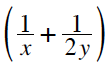2. ab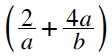3. 2x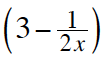4. What expression would go in the box to make the equation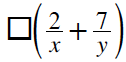= 2y + 7x true?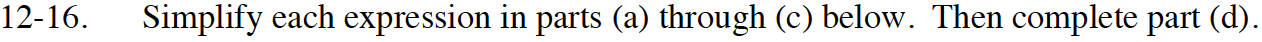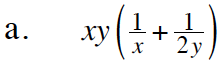Distribute the xy to both terms in the parentheses ( ).
Then simplify.

$\textit{y}+\frac{\textit{x}}{2}$

2b + 4a2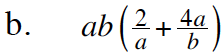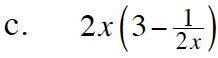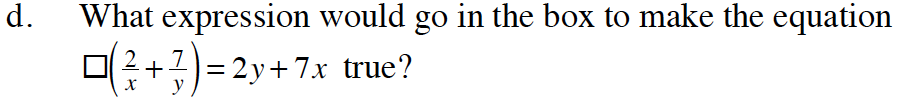See the previous parts of this problem for clues.